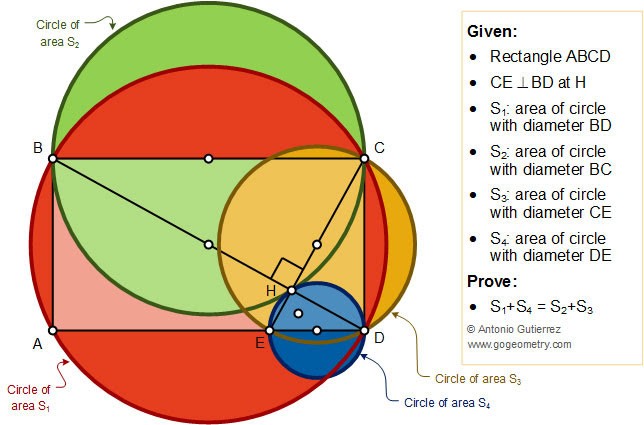## Sunday, June 9, 2019

### Geometry Problem 1439: Rectangle, Diagonal, Perpendicular, Circles, Areas

Geometry Problem. Post your solution in the comment box below.
Level: Mathematics Education, High School, Honors Geometry, College.

Details: Click on the figure below.#### 1 comment:

1.From Pythagoras,

CD^2 = CE^2 - DE^2 = BD^2 - BC^2 and so
BD^2 + DE^2 = BC^2 + CE^2

Hence S1 + S4 = S2 + S3

Sumith Peiris
Moratuwa
Sri Lanka